Question

Criticize the following incorrect statement:

“If an object is at rest and the total force on it is zero, it stays at rest. There can also be cases where an object is moving and keeps on moving without having any total force on it, but that can only happen when there’s no friction, like in outer space.”

There is nothing wrong in this statement.

according to newton first law of motion,

a body is in motion or rest tends to be in motion or rest unless an external force is applied.

So, like in vacuum , if body is in rest , it will remain in rest if force is not applied.

Same applied for body in motion, it will remain in motion if force is not applied

#### Earn Coins

Coins can be redeemed for fabulous gifts.

Similar Homework Help Questions
• ### Problem A 100 kg object that is at rest, pushes with a force of 250 Newtons,...

Problem A 100 kg object that is at rest, pushes with a force of 250 Newtons, moving it in a straight line at a distance of 15 meters along a horizontal floor with a coefficient of kinetic friction equal to 0.25. Calculate: a) the work of the applied force (WF) b) the magnitude of the weight (w) c) the magnitude of the kinetic friction force (Frk d) the work of the kinetic friction (WFrk) e) the total work (Wtotal) f...

• ### Which of the following is incorrect concerning Newton's First Law? I know that Newton's first law...

Which of the following is incorrect concerning Newton's First Law? I know that Newton's first law states an object at rest will remain at rest as long as the net force on the object is zero. I narrowed the questions down to three choices of the five: A) An object can not have a constant speed if the magnitude of net force is greater than zero. B) An object can not have a constant veloocity if the magnitude of net...

• ### understanding newton's law 1. An object cannot remain at rest unless which of the following holds? a. The net force act...

understanding newton's law 1. An object cannot remain at rest unless which of the following holds? a. The net force acting on it is zero. b. The net force acting on it is constant and nonzero. c. There are no forces at all acting on it. d. There is only one force acting on it. which one is the answer? there can be more than one answer.... 2. If a block is moving to the left at a constant velocity,...

• ### 5) An object is moving to the left at constant velocity. A constant force to the left is now applied to the object...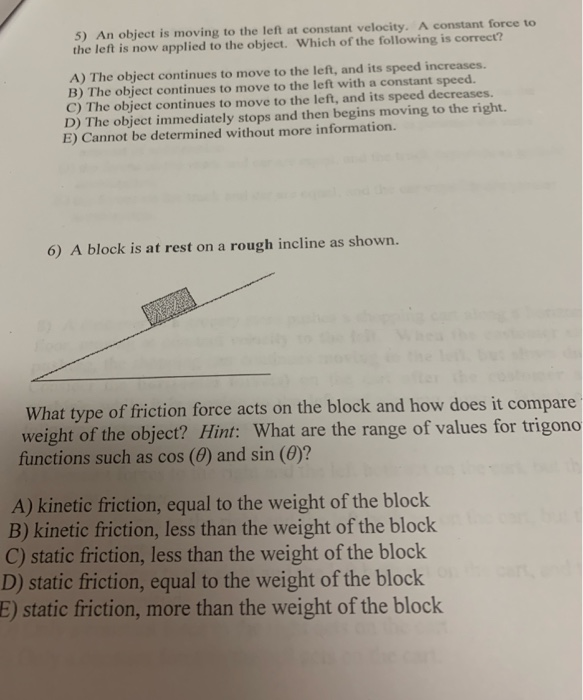5) An object is moving to the left at constant velocity. A constant force to the left is now applied to the object. Which of the following is correct? A) The object continues to move to the left, and its speed increases. B) The object continues to move to the left with a constant speed. C) The object continues to move to the left, and its speed decreases. D) The object immediately stops and then begins moving to the right...

• ### 6. Consider the equation Σ F-mv2/r for the total force exerted on an object in uniform...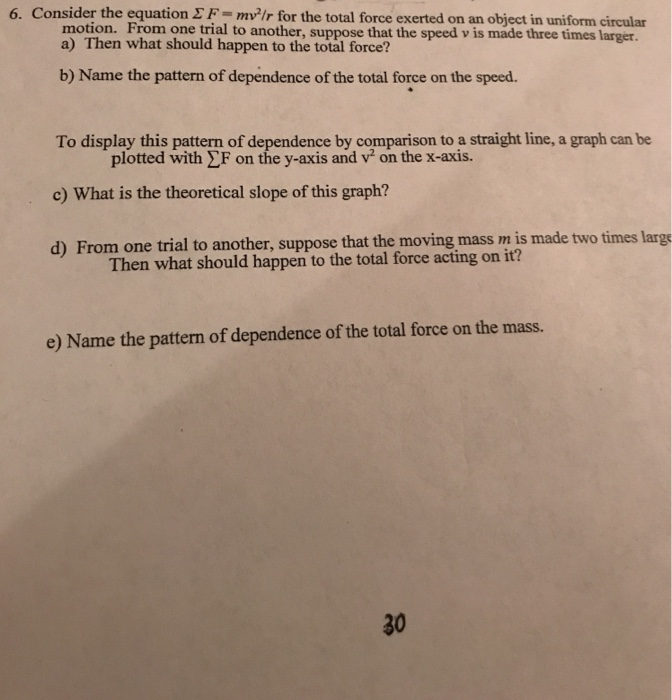6. Consider the equation Σ F-mv2/r for the total force exerted on an object in uniform circular motion. From one trial to a) Then what should happen to the total force? b) Name the pattern of dependence of the total force on the speed To display this pattern of dependence by comparison to a straight line, a graph can be c) What is the theoretical slope of this graph? d) From one trial to another, suppose that the moving mass...

• ### MULTIPLE CHOICE, Show your work in the space provided, Circle the correct answer 1. An object...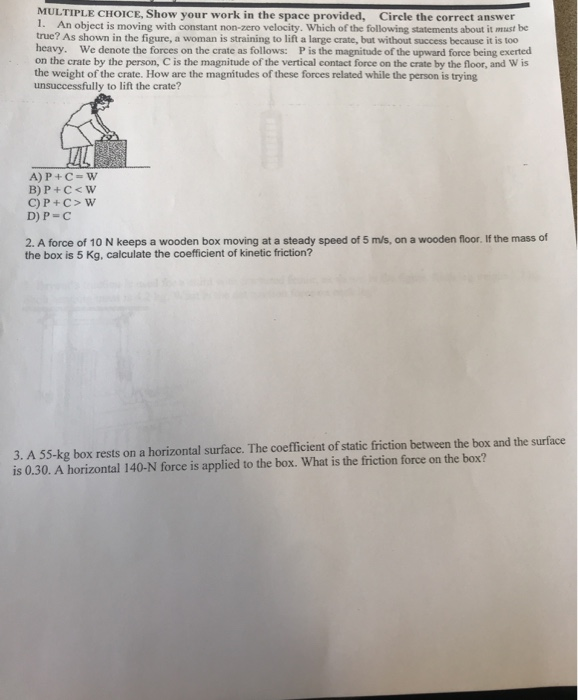MULTIPLE CHOICE, Show your work in the space provided, Circle the correct answer 1. An object is moving with constant non-zero velocity. Which of the following statements about it must be true? As shown in the figure, a woman is straining to lift a large crate, but without success because it is too heavy. We denote the forces on the crate as follows: P is the magnitude of the upward force being exerted on the crate by the person, C...

• ### When an object falls in Earth's gravitational field (think of a skydiver jumping from an airplane or a marble falling in a tank of oil)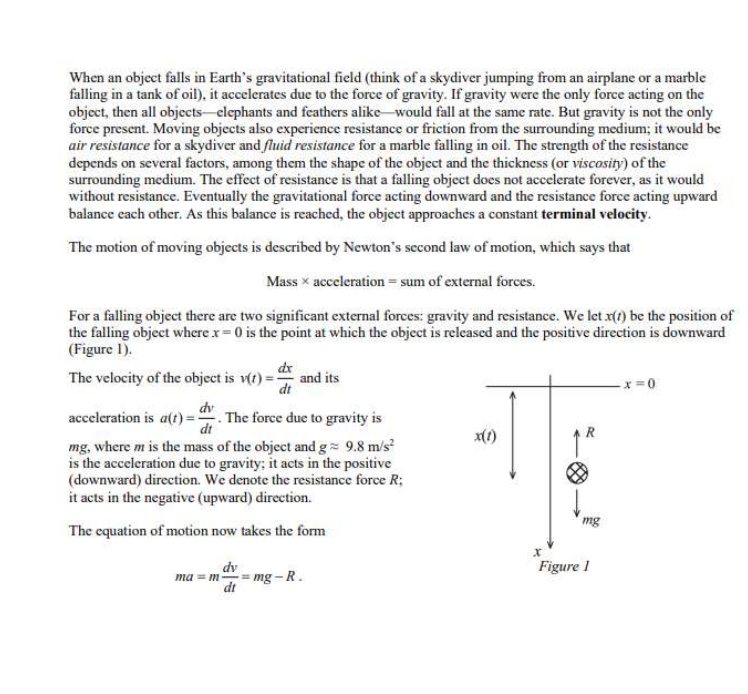When an object falls in Earth's gravitational field (think of a skydiver jumping from an airplane or a marble falling in a tank of oil), it accelerates due to the force of gravity. If gravity were the only force acting on the object, then all objects-elephants and feathers alike would fall at the same rate. But gravity is not the only force present. Moving objects also experience resistance or friction from the surrounding medium; it would be air resistance for...

• ### absolute C++ QUESTION 1 Which statement is incorrect? When an event occurs that cannot be managed...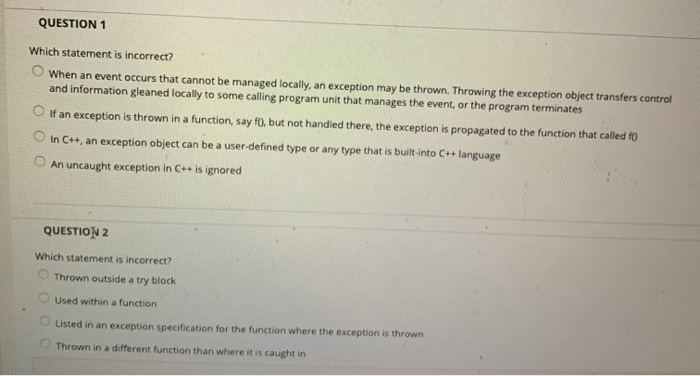absolute C++ QUESTION 1 Which statement is incorrect? When an event occurs that cannot be managed locally, an exception may be thrown. Throwing the exception object transfers control and information gleaned locally to some calling program unit that manages the event, or the program terminates If an exception is thrown in a function, sayf, but not handled there, the exception is propagated to the function that called fo In C++, an exception object can be a user-defined type or any...

• ### 7 An object of mass 2.00 kg starts at rest from the top of a tough...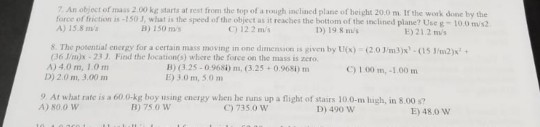7 An object of mass 2.00 kg starts at rest from the top of a tough inclned plane of height 20.0 m. If the work done by the force of friction as-150 3, what is the speed of the object as it reaches the botiom of the inclined plane? Use g- 1o.0 m/s A) 15.8 m B) 150 ros C) 12 2 ms D) 19 8 ms E) 21.2 ms S. The posential energy for a certain mass moving in...

• ### S/13495/quizzes/27508/take D Question 1 10 pts Which among the following statements is CORRECT? An object's weight...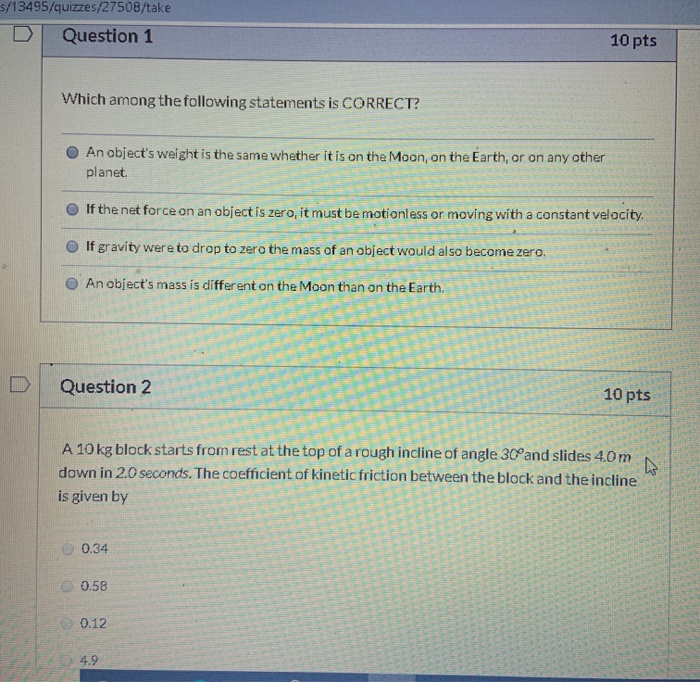S/13495/quizzes/27508/take D Question 1 10 pts Which among the following statements is CORRECT? An object's weight is the same whether it is on the Moon, on the Earth, or on any other planet If the net force on an object is zero, it must be motionless or moving with a constant velocity. If gravity were to drop to zero the mass of an object would also become zero. An object's mass is different on the Moon than on the Earth....